# Spectral theory of compact operators

Letbe a complex Banach space anda compact operator on. Then, the spectrum of, is countable and has no cluster points except, possibly,. Every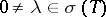is an eigenvalue, and a pole of the resolvent function. Let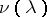be the order of the pole. For each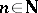,is closed, and this range is constant for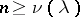. The null space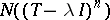is finite dimensional and constant for. The spectral projection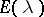(the Riesz projector, see Riesz decomposition theorem) has non-zero finite-dimensional range, equal to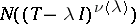, and its null space is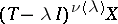. Finally,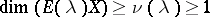.
The respective integersand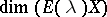are called the index and the algebraic multiplicity of the eigenvalue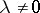.